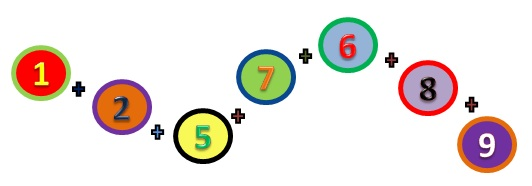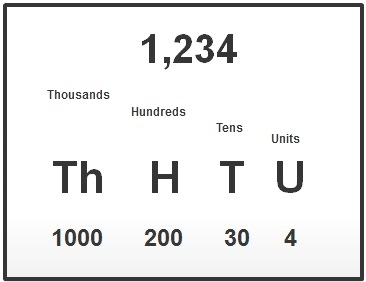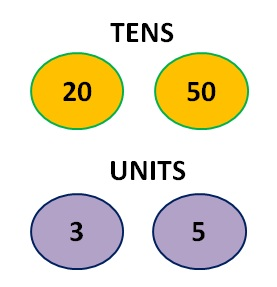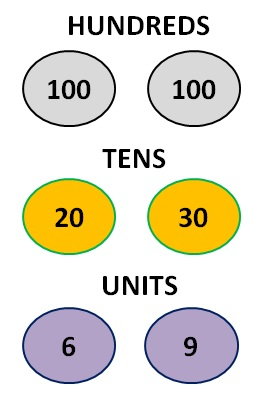Look at the examples below before you attempt any of the exercises. It is important to understand how numbers are placed into units, tens, hundreds and thousands.

## Example 1

5 + 5 =?

5 + 5 = 10 enter 0 in the units column and carry 1 to the tens column.

ThHTU
5
5
10
1

+

## Example 2

82 + 37 =?

1. 2 + 7 = 9 enter 9 in the units column.

2. 8 + 3 = 11 enter 1 in the tens column and carry 1 over to the hundreds column.

ThHTU
82
37
119

+

## Example 3

145 + 935 =?

1. 5 + 5 = 10 enter 0 in the units column
and carry 1 over to the tens column.

2. 4 + 3 = 7 plus the 1 carried over = 8
enter 8 in the tens column.

3. 9 + 1 = 10 enter 0 in the hundreds
column and carry 1 over to the thousands
column.

ThHTU
145
935
1 080
1

+

# VIDEO

Have a look at the demonstration on the video to enhance your learning.## Example 1

1. Add the tens 20 + 50 = 70
2. Add the units 3 + 5 = 8
3. Then add up the results 70 + 8

Total= 78## Example 2

1. Add the hundreds 100 + 100 = 200
2. Add the tens 20 + 30 = 50
3. Add the units 6 + 9 = 15
4. Then add up the results 200 + 50 + 15

Total = 265Now try the Exercises!